# Ordinary magnetoresistance from first-principle calculation

Magnetoresistance (MR) is the change of electrical resistance in an applied magnetic field. Magnetoresistance is commonly defined as MR(B) = [ρ(B) − ρ(0)]/ρ(0), where ρ(0) and ρ(B) are electrical resistivities in zero field and in applied magnetic field B, respectively. Positive MR typically occurs in metals, semiconductors, and semimetals, while negative MR is seen in magnetic materials and some topological materials recently. MR is a relatively weak effect in most nonmagnetic compounds, being characterized by quadratic field dependence in low fields that saturates to a magnitude of a few percent in the case of metals. There are many kinds of MR effects due to different mechnisims. In this review, we will only focus on ordinary magnetoresistance of non-interacting, non-magnetic metals and semimetals. Even thought, there are several interesting phenomena like extreme MR (XMR), anistropic MR. There are many experiments studying those effects on different materials, such as Type-II Weyl semimetals WTe2, MoTe2, and WP2, PdCoO2, PtSn4, NbSb2, LaSb, YSb, MoAs2, NbAs2, TaAs2, TmSb, α-WP2 etc. The corresponding references can be found in PhysRevB.99.035142.

We have several assumptions. 1). The Fermi surface would not be affected by the magnetic field. This is called rigid band approximation. 2). Relaxation-time approximation. 3). Berry phase and Berry curvature are not considered.

Based on above assumptions and according to the Boltzmann transport theory,The conductivity tensor σ is calculated in presence of an applied magnetic field as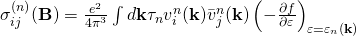where e is the electron charge, n is the band index,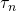is the relaxation time of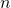-th band that is assumed to be independent on the wave vector,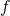is the Fermi-Dirac distribution,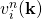is the velocity defined by the gradient of band energy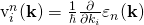and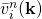is the weighted average of velocity over the past history of the charge carrier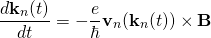with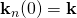. The trajectory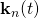can be obtained by integrating the above equation. As a consequence,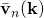can be calculated as the weighted average of the velocities along the trajectory.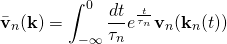In this semiclassical picture, the Lorentz force does no work on charge carriers since it is perpendicular to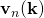, and therefore energy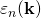remains constant as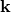evolves in time, implying that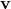is normal to the constant energy surface, and consequently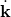is tangential to it. Sinceis also perpendicular to magnetic field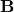, it follows that thevector traces out an orbit which is a cross-section of the Fermi surface by a plane normal to.

In the implementation, usually, we put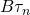together. Reformulate as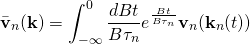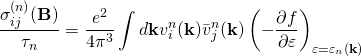References

1. R. G. Chambers, Electrons in Metals and Semiconductors (Chapman and Hall, London, 1990).

2. N.W.Ashcroft and N.D.Mermin, Solid state physics (Saunders college publishing)

3. Yi Liu, Hai-Jun Zhang, and Yugui Yao, Ab initio investigation of magnetic transport properties by Wannier interpolation, Phys. Rev. B 79, 245123 (2009)

4. ShengNan Zhang, QuanSheng Wu, Yi Liu, and Oleg V. Yazyev, Magnetoresistance from Fermi surface topology, Phys. Rev. B 99, 035142 (2019)

Visiting: 2,852 views